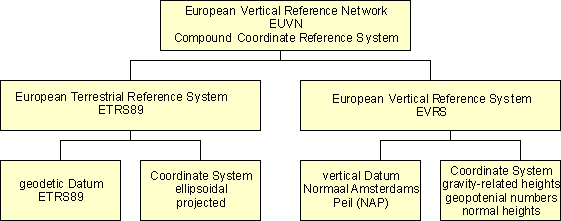﻿ CRS-EU   Definition of a CRS

# Definition of a Coordinate Reference System (CRS)

A Coordinate Reference System contains two different elements - the datum and the coordinate system.

• The datum defines how the CRS is related to the earth (position of the origin, the scale and the orientation of coordinate axis) e.g. ED50, ETRS89 and the coordinate system describes how the coordinates expressed in the datum e.g. as cartesian coordinates, ellipsoidal coordinates or coordinates of a map projection e.g. UTM.

The datum can be a geodetic datum, a vertical datum or a engineering / local datum.

The coordinate system is the mathematical part of Coordinate Reference System is a set of rules e.g. projection equations for specifying how the coordinates are to be assigned to the points.Schema of CRS Defintion

The horizontal and vertical components of the description of a position in the space may sometimes come from different Coordinate Reference Systems. This shall be handled by a compound Coordinate Reference System (CCRS). The compound Coordinate Reference System describes the position by two independent Coordinate Reference Systems. An European spatial reference system could be described as a compound Coordinate Reference System. An example is shown in the figure.Example of compound CRS